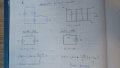# Buck Converter Simulation in Python

#### VektorElektor

Joined Jun 21, 2023
1
Hey everybody, first time here!

As a personal project I am implementing a Buck Converter simulation in Python. My basic idea is to give a constant Vs supply and simulate the switch on/switch off steps in each switching period, calculate the increase/decrease in inductor current and calculate an output voltage Vo. The duty cycle is an input and the output voltage Vo should be a result.

I started with a Vo = 0 and Vs = 230V. I expected the current in the inductor to gradually grow until it would reach steady-state operation. However, I am incorrectly updating the Vo, so it remains constant all the way and the current keep growing and growing forever. I based switch on and switch off steps on my notes from the picture:So in my simulation I'm following this process for each step (switch on for instance):
- Calculate the voltage drop in the inductor VL = Vs - Vo
- Calculate the current increase as /\iL = ( VL/ L ) * t_on
- Update the inductor current as iL = iL_past + /\iL

However, since the idea of a Buck Converter is to downstep the voltage, I'm also updating Vo like so:
- VL = L * ( /\iL / t_on )
- Vo = Vs - VL

This is where the Vo does not get updated and remains constant. I understand that I'm using the same formula to calculate iL from Vo and to calculate Vo from iL, so it's obviously redundant and wrong. Can someone help me rethink this? I'm also worried that a capacitor would be necessary in the output side, parallel to Vo.